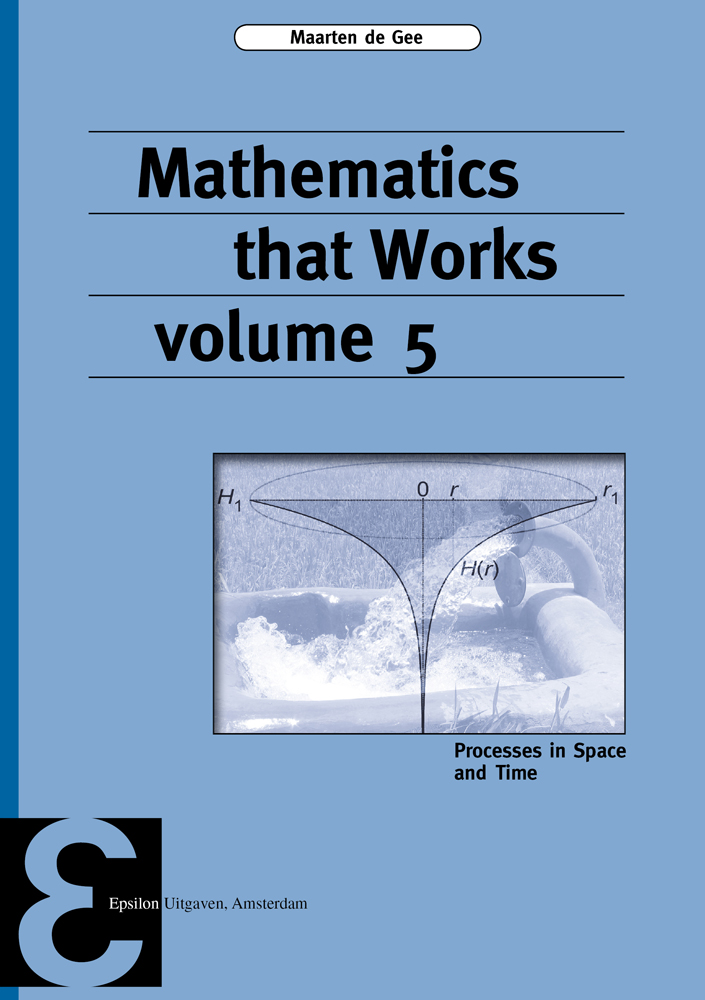ePUB ebook

niet beschikbaar

PDF ebook

niet beschikbaar

# 5

### Maarten de Gee • Boek • paperback

• Samenvatting
From ancient times, mathematics has developed side-by-side with physics; only in the last two hundred years they came apart as different branches of science. Extensive use of mathematics in other sciences, such as biology, chemistry, economics, pharmacology, environmental and social sciences is even more recent. Nowadays, knowledge of mathematical techniques is indispensable also for students and researchers in these fields. The series of textbooks “Mathematics that Works” presents mathematical methods with a sharp focus on applicability. There is an ample supply of worded-out examples and instructive exercises. The techniques are illustrated in applications from various branches of science; here, the connection between the mathematical method and the application is equally important as the mathematics itself. Thus the way the students digest the matter is aimed at practical usability within their own field, and skills take priority over the mathematical abstraction level. By connecting mathematics to its applications the usage of mathematics in the applied sciences is facilitated. This fifth volume treats processes with a spatial component. We start with differentiation and integration of functions with several variables, and we conclude with processes that can be modelled with partial differential equations. The reader is assumed to be familiar with calculus and linear algebra (for example from the volumes 1, 2, and 3 of this series) and ordinary differential equations (volume 4). The level of difficulty is higher than in the previous volumes; mathematical techniques stack on each other, the applications are more advanced, and the mathematical modelling has more emphasis.
• Productinformatie
Binding : Paperback
Distributievorm : Boek (print, druk)
Formaat : 170mm x 240mm
Aantal pagina's : 496
Uitgeverij : Epsilon Uitgaven
ISBN : 9789050411707
Datum publicatie : 09-2018
• Inhoudsopgave
niet beschikbaar
• Reviews (0 uit 0 reviews)

# € 39,00niet beschikbaarniet beschikbaar

Voor 21:00 besteld, morgen in huis!
Veilig betalen14 dagen bedenktermijn
Delen

niet beschikbaar

×
Contact